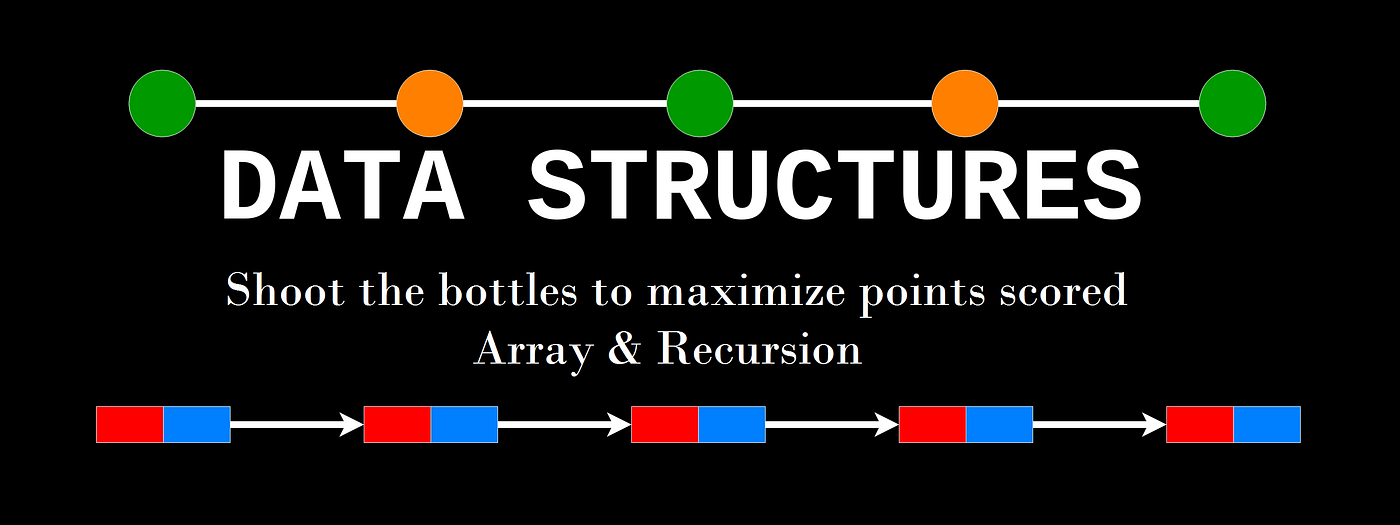# Data structures: Shoot the bottles to maximize points scored — Array & RecursionShoot the bottles to maximize points scored — Array & Recursion

Data structure questions are quite common to be asked in the interviews these days. The expectation from you is to come up not only with a feasible approach, but also to write a working code for the same.

Here is a little brain-teaser for you.

Problem Statement:
There are n bottles, each having a certain number of points associated with it. Once you shoot the bottle, you score those points. You have k bullets to shoot k bottles. Determine the maximum points which can be scored.
Constraint: You have to leave a distance greater than or equal to reloading gap between two shots.

Example:
Bottles: {10, 100, 10, 10, 10, 100, 10, 10, 10, 100}
Bullets: 3 (number of shots)
Reloading gap: 1 (indices of bottles which are shot should differ at least by 1)

The first thing which comes to mind is that we have to consider all possible solution sets, and then pick the maximum from it. Further, since the reloading gap is not fixed. We are given with a minimum gap, which means it can be more if it gives us more points.

Approach:
The first approach which comes to mind a recursion based approach. At each bottle, we have a chance to either shoot or leave the bottle.

If we shoot the bottle, the following happens:
1. The bullet count decrease by one.
2. The sum increase by the number of points the bottle had.
3. Next possible hit is next to current index plus the reloading gap.

If we leave the bottle, the following happens:
1. The bullet count remains same.
2. The sum remains same.
3. Next possible hit is the immediate next index.

Terminating condition:
The most important thing in a recursion code is it’s terminating condition. Our code will terminate here is there are no bullets left.

Additionally, we have to keep in mind that the next possible hit index doesn’t go out of range.

Keeping all the above in mind. Here is a working recursive solution for this problem, with each section explained:

Driver code (with test cases):

`public static void main(String str[]) {    int[] points;    points = new int[]{10, 10, 10, 10, 10, 10, 10, 10, 10, 10};    assertEqual(calculateMaxScore(points, 5, 1), 50);    points = new int[]{10, 100, 10, 10, 10, 100, 10, 10, 10, 100};    assertEqual(calculateMaxScore(points, 3, 1), 300);    points = new int[]{10, 100, 500, 10, 10, 100, 500, 10, 10, 100};    assertEqual(calculateMaxScore(points, 3, 1), 1100);    points = new int[]{10, 5, 11, 8, 7, 5, 2, 9, 13, 1, 12, 8, 4, 8, 9, 4, 12, 11, 8, 7, 5, 12, 8,            5, 9, 4, 7, 3, 11, 10, 5, 3, 7, 10, 9, 8, 4, 7, 10, 9, 12, 4, 6, 3, 8, 6, 9, 6, 2, 9};    assertEqual(calculateMaxScore(points, 10, 2), 108);}public static void assertEqual(Object o1, Object o2) {    if (o1.equals(o2))        System.out.println("Pass");    else        System.out.println("Fail");}`

Implementation:
Here is the working code for the above approach:

`static int count = 0;public static int calculateMaxScore(int[] points, int bullets, int reloadingGap) {    count=0;    int result = getScore(points, bullets, reloadingGap, 0, 0);    System.out.println(count);    return result;}private static int getScore(int[] points, int bullets, int reloadingGap, int startIndex, int sum) {    count++;    if(bullets == 0) {        return sum;    }    int sum1=0;    // bottle shot    if(startIndex <= points.length-1) {        sum1=sum+points[startIndex];            if(startIndex + reloadingGap + 1 <= points.length-1){                sum1 = getScore(points, bullets-1, reloadingGap, startIndex+reloadingGap+1, sum1);            }    }    // bottle left    int sum2=0;    if(startIndex+1 <= points.length-1) {            sum2 = getScore(points, bullets, reloadingGap, startIndex+1, sum);    }    if(sum1==0 && sum2==0) {        return sum;    }    return Math.max(sum1, sum2);}`

Output:
143
Pass
122
Pass
122
Pass
184715259
Pass

Complexity Analysis:
Since each of the above bottle can either be shot or left, the complexity runs exponential. Here is a quick gauge on same by capturing the number of recursive call in each of the test cases:

Length of array: 10
Recursive calls: 143

Length of array: 10
Recursive calls: 122

Length of array: 10
Recursive calls: 122

Length of array: 50
Recursive calls: 184715259

The first three run counts are near about, as number reloading gap is same. Notice the run counts when the number of bottles increase. Also, if you reduce the reloading gap to zero, the run count will come to 1024 (2 power 10), which is exponential!

In the next article, we will discuss about a Dynamic Programming version of this problem. We will also analyze the impact on run count with that approach. Stay tuned.

Here is where we can connect to discuss more: